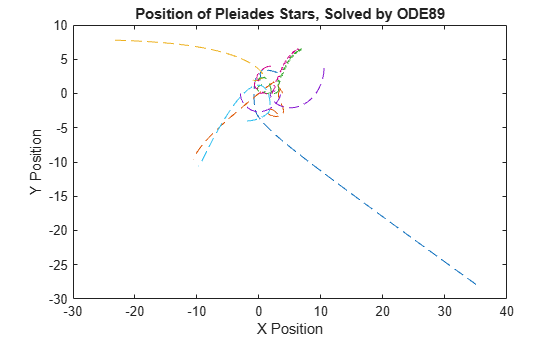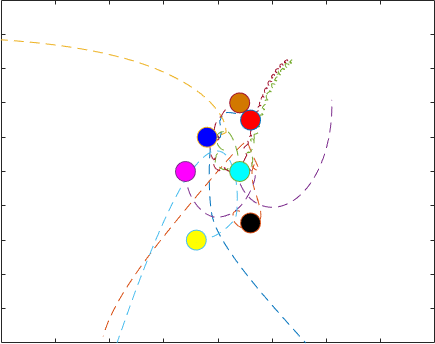# Solve Celestial Mechanics Problem with High-Order Solvers

This example shows how to use `ode78` and `ode89` to solve a celestial mechanics problem that requires high accuracy in each step from the ODE solver for a successful integration. Both `ode45` and `ode113` are unable to solve the problem using the default error tolerances. Even with more stringent error thresholds, `ode89` performs best on the problem due to the high accuracy of the Runge-Kutta formulas it uses in each step.

### Problem Description

The Pleiades problem is a celestial mechanics problem describing the gravitational interactions of seven stars . This cluster of stars is also referred to as The Seven Sisters, and it is visible to the human eye in the night sky due to its proximity to Earth . The system of equations describing the motion of the stars in the cluster consists of 14 nonstiff second-order differential equations, which produce a system of 28 equations when rewritten in first-order form.

Newton's second law of motion relates the force applied to a body to its rate of change in momentum over time,

${\mathit{F}}_{\mathit{i}}=\frac{\mathrm{d}}{\mathrm{d}\mathit{t}}{\mathit{p}}_{\mathit{i}}$.

The momentum (${\mathit{p}}_{\mathit{i}}={\mathit{m}}_{\mathit{i}}{\mathit{v}}_{\mathit{i}}$) has separate x- and y-components. At the same time, the universal law of gravitation describes the force working on body i from body j as

${\mathit{F}}_{\mathrm{ij}}=\mathit{g}\frac{{\mathit{m}}_{\mathit{i}}{\mathit{m}}_{\mathit{j}}}{{‖{\mathit{p}}_{\mathit{i}}-{\mathit{p}}_{\mathit{j}}‖}^{2}}{\mathit{d}}_{\mathrm{ij}}$.

The term ${\mathit{d}}_{\mathrm{ij}}=\frac{{\mathit{p}}_{\mathit{j}}-{\mathit{p}}_{\mathit{i}}}{‖{\mathit{p}}_{\mathit{j}}-{\mathit{p}}_{\mathit{i}}‖}$ gives the direction of the distance between the bodies, and the masses of the bodies are ${\mathit{m}}_{\mathit{i}}=\mathit{i}$ for $\mathit{i}=1,2,...,7$. For a system with many bodies, the force applied to any individual body is the sum of its interactions with all others, so

`${\mathit{F}}_{\mathit{i}}=\sum _{\mathit{i}\ne \mathit{j}}{\mathit{F}}_{\mathrm{ij}}.$`

Setting the gravitational constant $\mathit{g}$ equal to 1 and solving yields a system of second-order equations describing the evolution of the x- and y-components over time.

`${{{\mathit{x}}_{\mathit{i}}}^{\prime }}^{\prime }={\mathit{f}}_{\mathit{i}}\left(\mathit{x},\mathit{y}\right)=\sum _{\mathit{j}\ne \mathit{i}}\frac{{\mathit{m}}_{\mathit{j}}\left({\mathit{x}}_{\mathit{j}}-{\mathit{x}}_{\mathit{i}}\right)}{{\mathit{r}}_{\mathrm{ij}}^{\text{\hspace{0.17em}}3/2}},$`

`${{{\mathit{y}}_{\mathit{i}}}^{\prime }}^{\prime }={\mathit{h}}_{\mathit{i}}\left(\mathit{x},\mathit{y}\right)=\sum _{\mathit{j}\ne \mathit{i}}\frac{{\mathit{m}}_{\mathit{j}}\left({\mathit{y}}_{\mathit{j}}-{\mathit{y}}_{\mathit{i}}\right)}{{\mathit{r}}_{\mathrm{ij}}^{\text{\hspace{0.17em}}3/2}},$`

where ${\mathit{r}}_{\mathrm{ij}}=\sqrt{{\left({\mathit{x}}_{\mathit{i}}-{\mathit{x}}_{\mathit{j}}\right)}^{2}+{\left({\mathit{y}}_{\mathit{i}}-{\mathit{y}}_{\mathit{j}}\right)}^{2}}$. Because these two equations apply for each of the seven stars in the system, 14 second-order differential equations ($\mathit{i}=1,2,...,7\right)$ describe the entire system.

The initial conditions for the system, as given in , are:

`$\left\{\begin{array}{cc}{x}_{0}& =\left(3,3,-1,-3,2,-2,2\right)\\ {y}_{0}& =\left(3,-3,2,0,0,-4,4\right)\\ {x}_{0}^{\prime }& =\left(0,0,0,0,0,1.75,-1.5\right)\\ {y}_{0}^{\prime }& =\left(0,0,0,-1.25,1,0,0\right)\end{array}$`

To solve this system of ODEs in MATLAB®, you must code the equations into a function before calling the solvers `ode78` and `ode89`. You can either include the required functions as local functions at the end of a file (as done here), or save them as separate, named files in a directory on the MATLAB path.

### Code Equations

The ODE solvers in MATLAB require equations to be written in first-order form, ${\mathit{q}}^{\prime }=\mathit{u}\left(\mathit{t},\mathit{q}\right)$. For this problem, the system of equations can be rewritten in first-order form using the substitutions $\mathit{w}={\mathit{x}}^{\prime }$ and $\mathit{z}={\mathit{y}}^{\prime }$. With these substitutions, the system contains 28 first-order equations organized into four groups of seven equations:

$\left[\begin{array}{c}{x}_{i}^{\prime }\\ {y}_{i}^{\prime }\\ {w}_{i}^{\prime }\\ {z}_{i}^{\prime }\end{array}\right]=\left[\begin{array}{c}{w}_{i}\\ {z}_{i}\\ {f}_{i}\left(x,y\right)\\ {h}_{i}\left(x,y\right)\end{array}\right]$.

The solution vector produced by solving the system has the form

`$\mathit{q}=\left[\begin{array}{c}{\mathit{x}}_{\mathit{i}}\\ {\mathit{y}}_{\mathit{i}}\\ {\mathit{w}}_{\mathit{i}}\\ {\mathit{z}}_{\mathit{i}}\end{array}\right].$`

Therefore, writing the original equations in terms of the solution vector $\mathit{q}$ yields

`$\left[\begin{array}{c}{{\mathit{x}}_{\mathit{i}}}^{\prime }\\ {{\mathit{y}}_{\mathit{i}}}^{\prime }\\ {{\mathit{w}}_{\mathit{i}}}^{\prime }\\ {{\mathit{z}}_{\mathit{i}}}^{\prime }\end{array}\right]=\left[\begin{array}{c}\left({\mathit{q}}_{15},{\mathit{q}}_{16},...,{\mathit{q}}_{21}\right)\\ \left({\mathit{q}}_{22},{\mathit{q}}_{23},...,{\mathit{q}}_{28}\right)\\ {\mathit{f}}_{\mathit{i}}\left(\mathit{x},\mathit{y}\right)\\ {\mathit{h}}_{\mathit{i}}\left(\mathit{x},\mathit{y}\right)\end{array}\right],$`

where$\mathit{x}=\left({\mathit{q}}_{1},{\mathit{q}}_{2},...,{\mathit{q}}_{7}\right)$ and $\mathit{y}=\left({\mathit{q}}_{8},{\mathit{q}}_{9},...,{\mathit{q}}_{14}\right)$. With the equations written in the first-order form ${\mathit{q}}^{\prime }=\mathit{u}\left(\mathit{t},\mathit{q}\right)$, you can now write a function that calculates the components during each time step of the solution process. A function that codes this system of equations is:

```function dqdt = pleiades(t,q) x = q(1:7); y = q(8:14); xDist = (x - x.'); yDist = (y - y.'); r = (xDist.^2+yDist.^2).^(3/2); m = (1:7)'; dqdt = [q(15:28); sum(xDist.*m./r,1,'omitnan').'; sum(yDist.*m./r,1,'omitnan').']; end ```

In this function, the $\mathit{x}$ and $\mathit{y}$ values are extracted directly from the solution vector $\mathit{q}$, as are the first 14 components of the output. Then, the function uses the position values to calculate the distances between all seven stars, and these distances are used in the code for ${\mathit{f}}_{\mathit{i}}\left(\mathit{x},\mathit{y}\right)$ and ${\mathit{h}}_{\mathit{i}}\left(\mathit{x},\mathit{y}\right)$.

### Set Optional Parameters

Use the `odeset` function to set the value of several optional parameters:

• Specify stringent error tolerances of `1e-13` and `1e-15` for the relative and absolute error tolerances, respectively.

• Turn on the display of solver statistics.

`opts = odeset("RelTol",1e-13,"AbsTol",1e-15,"Stats","on");`

### Solve System of Equations

Create a column vector with the initial conditions and a time vector with regularly spaced points in the range $\left[0,15\right]$. When you specify a time vector with more than two elements, the solver returns solutions at the time points you specify.

```init = [3 3 -1 -3 2 -2 2 ... 3 -3 2 0 0 -4 4 ... 0 0 0 0 0 1.75 -1.5 ... 0 0 0 -1.25 1 0 0]'; tspan = linspace(1,15,200);```

Now, solve the equations using both `ode78` and `ode89` by specifying the equations, time span, initial values, and options. Use `tic` and `toc` to time each solver for comparison (note that timings can differ depending on your computer).

```tic [t78,q78] = ode78(@pleiades,tspan,init,opts);```
```14963 successful steps 549 failed attempts 201899 function evaluations ```
`toc`
```Elapsed time is 6.007655 seconds. ```
```tic [t89,q89] = ode89(@pleiades,tspan,init,opts);```
```4181 successful steps 56 failed attempts 68726 function evaluations ```
`toc`
```Elapsed time is 3.102789 seconds. ```

The output indicates that `ode89` is best suited for solving the problem, because it is faster and has fewer failed steps.

### Plot Solution Curves

The first 14 components of `q89` contain the x and y positions for each of the seven stars, as obtained by `ode89`. Plot these solution components to see the trajectories of all the stars over time.

```plot(q89(:,1),q89(:,8),'--',... q89(:,2),q89(:,9),'--',... q89(:,3),q89(:,10),'--',... q89(:,4),q89(:,11),'--',... q89(:,5),q89(:,12),'--',... q89(:,6),q89(:,13),'--',... q89(:,7),q89(:,14),'--') title('Position of Pleiades Stars, Solved by ODE89') xlabel('X Position') ylabel('Y Position')```### Create Animation of Results

Because the trajectories of the stars overlap considerably, a better way to visualize the results is to create an animation showing the stars move over time. The function `AnimateOrbits`, included as a local function at the end of this example, accepts the output from a solver for this problem and creates an animated GIF file in the current folder that shows the stars move along their trajectories.

For example, you can generate an animation with the output from the `ode89` solver using the command

```AnimateOrbits(t89,q89); ```

The following is a sample output GIF.### References

 Hairer, E., et al. Solving Ordinary Differential Equations I: Nonstiff Problems. 2nd rev. ed, Springer, 2009.

### Local Functions

This section includes the local functions called by the ODE solver to calculate the solution. Alternatively, you can save these functions as their own files in a directory on the MATLAB path.

```function dqdt = pleiades(t,q) x = q(1:7); y = q(8:14); xDist = (x - x.'); yDist = (y - y.'); r = (xDist.^2+yDist.^2).^(3/2); m = (1:7)'; dqdt = [q(15:28); sum(xDist.*m./r,1,'omitnan').'; sum(yDist.*m./r,1,'omitnan').']; end %----------------------------------------------------------------- function AnimateOrbits(t,q) for k = 1:length(t) plot(q(:,1),q(:,8),'--',q(:,2),q(:,9),'--',... q(:,3),q(:,10),'--',q(:,4),q(:,11),'--',... q(:,5),q(:,12),'--',q(:,6),q(:,13),'--',... q(:,7),q(:,14),'--') hold on xlim([-20 20]) ylim([-10 10]) sz = 15; plot(q(k,1),q(k,8),'o','MarkerSize',sz,'MarkerFaceColor','r') plot(q(k,2),q(k,9),'o','MarkerSize',sz,'MarkerFaceColor','k') plot(q(k,3),q(k,10),'o','MarkerSize',sz,'MarkerFaceColor','b') plot(q(k,4),q(k,11),'o','MarkerSize',sz,'MarkerFaceColor','m') plot(q(k,5),q(k,12),'o','MarkerSize',sz,'MarkerFaceColor','c') plot(q(k,6),q(k,13),'o','MarkerSize',sz,'MarkerFaceColor','y') plot(q(k,7),q(k,14),'o','MarkerSize',sz,'MarkerFaceColor',[210 120 0]./255) hold off drawnow M(k) = getframe(gca); im{k} = frame2im(M(k)); end filename = "orbits.gif"; for idx = 1:length(im) [A,map] = rgb2ind(im{idx},256); if idx == 1 imwrite(A,map,filename,'gif','LoopCount',Inf,'DelayTime',0); else imwrite(A,map,filename,'gif','WriteMode','append','DelayTime',0); end end close all end```